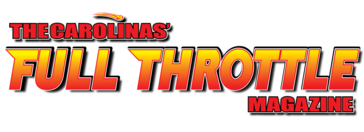# Welcome To The Carolinas' Full Throttle Magazine Cruisin' Calendar Weekly Update For June 7-11, 2018

If you want to see your event here or know of an event that should be posted here,
please e-mail the flyer/info to Lincoln@FTCarolinas.com.
Please include the date, start location (street address, city, state), any directions, and a contact phone number.

Please do not hesitate to call 336-885-5400 if I can help in any way.

Mark Infield
Editor / Owner
The Carolinas'
Full Throttle Magazine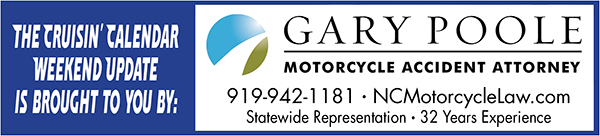Click Here To Visit Their Website!
 table div table+table+table+table div table{width:100%;padding:0}table div table+table+table+table div table img{width:96.23%;padding:0;float:none}table div table+table+table+table div table td{width:100%;padding:0 1.88% 18px}/* styles */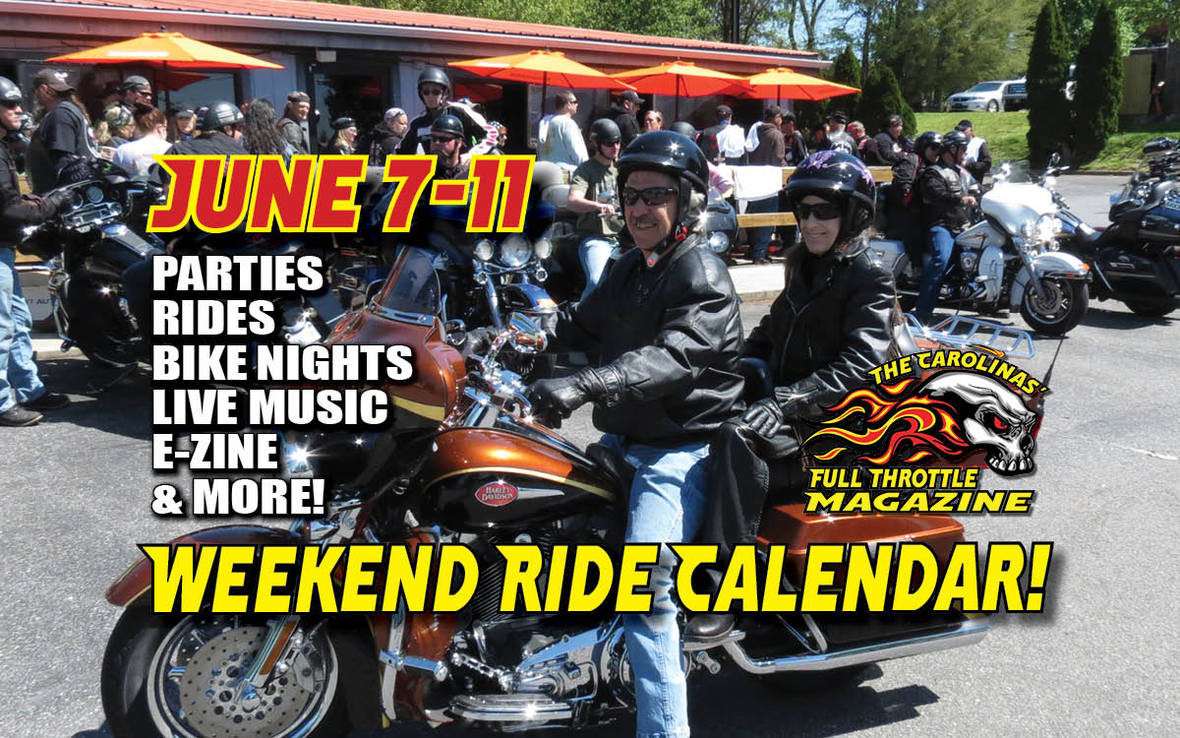table div table+table+table+table+table+table+table div table{width:100%;padding:0}table div table+table+table+table+table+table+table div table img{width:96.23%;padding:0;float:none}table div table+table+table+table+table+table+table div table td{width:100%;padding:0 1.88% 18px}/* styles */# Here Is What's Going On This Weekend...

 table div table+table+table+table+table+table+table+table+table div table{width:100%;padding:0}table div table+table+table+table+table+table+table+table+table div table img{width:96.23%;padding:0;float:none}table div table+table+table+table+table+table+table+table+table div table td{width:100%;padding:0 1.88% 18px}/* styles */# THURSDAY, JUNE 7

 /* styles */ JUN 7 BIKE NIGHT Hometown Harley-Davidson 2300 Elaine’s Way, Winterville, NC. Live music. www.hometownharleydavidson.com 252-439-1345. JUN 7 BIKE SHOW Smokin Harley-Davidson 3441 Myer Lee Dr., Winston-Salem, NC. 6-9, Reg between 6-8. Cash prize. 336-722-3106, www.smokinharley.com.

# THURSDAY-SATURDAY, JUNE 7-9

 /* styles */ JUN 7-9 SOUTH CENTRAL BIKE FEST Chase City, VA. Music, rides, camping, bikini bike wash, rodeo events. Benefits The Barksdale Cancer Foundation. www.southcentalbikefest.com Lee 434-210-1503.

# THURSDAY-SUNDAY, JUNE 7-10

 /* styles */ JUN 7-10 2018 HOG HAPPENIN' BIKE RALLY Hickory American Legion Fairgrounds 1 American Legion Ave., Newton, NC. Live music, gator girls, bike build competition, vendors, campsites available, daily 50/50, motorcycle pull, jello wrestling, swap meet, Blue Ridge rides, bike show, amateur BBQ competition, food. www.hoghappenin.org Brad “Rowdy” Beal 704-740-6659.
 table div table+table+table+table+table+table+table+table+table+table+table+table+table+table+table+table div table td,table.module-15{width:100%;padding:0}table div table+table+table+table+table+table+table+table+table+table+table+table+table+table+table+table div table{width:100%;float:none;margin-left:auto;margin-right:auto;padding:0}table div table+table+table+table+table+table+table+table+table+table+table+table+table+table+table+table div table a{border:0 none;text-decoration:none}table div table+table+table+table+table+table+table+table+table+table+table+table+table+table+table+table div table img{width:100%!important;border:0 none;text-decoration:none}/* styles */

# FRIDAY, JUNE 8

 /* styles */ JUN 8 CLASSIC CAR CRUISE-IN Ted’s Kickin’ Chicken 4695 S Main St, Winston-Salem NC 5p to 8p, free hot dogs for classic car drivers 336-650-0290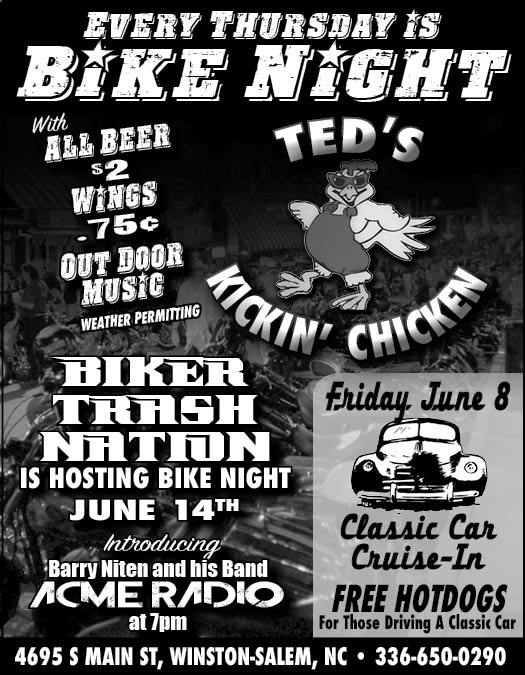https://www.facebook.com/tedssouthmain/

# SATURDAY, JUNE 9

 /* styles */ JUN 9 CROSSPOINTE CHURCH MOTORCYCLE RUN FOR THE MILITARY Crosspointe Church 7030 Rockfish Rd., Fayetteville, NC. Reg 9:30 KSU 10:30 \$15/bike \$20/pass. sappito@gmail.com Shane Sapp 910-850-6799. JUN 9 4TH ANNUAL JUNE JAMR Tobacco Road Harley-Davidson 1126 S. Saunders St., Raleigh, NC. Reg 9:30-11 KSU 12:30, \$25/bike or car \$10/pass, door prizes, escorted ride, 50/50, silent auction. Benefits Foundation for Sarcoidosis Research, Faith FWB Church and Kitty Askins Hospice Center of Goldsboro. www.thejunejamr.org thejunejamr@gmail.com 919-922-1106. JUN 9 AMERICANS ASSISTING VETERANS INAUGURAL RIDE Down Home Harley-Davidson 2215 W Hanford Rd., Burlington, NC. Reg 9:30-10:30 KSU 11, food, music, drawings, \$15/sgl \$5/pass. Money raised goes directly to assisting our Veterans. Mindy Smith 336-675-1547.
 table div table+table+table+table+table+table+table+table+table+table+table+table+table+table+table+table+table+table+table+table+table+table div table td,table.module-21{width:100%;padding:0}table div table+table+table+table+table+table+table+table+table+table+table+table+table+table+table+table+table+table+table+table+table+table div table{width:100%;float:none;margin-left:auto;margin-right:auto;padding:0}table div table+table+table+table+table+table+table+table+table+table+table+table+table+table+table+table+table+table+table+table+table+table div table a{border:0 none;text-decoration:none}table div table+table+table+table+table+table+table+table+table+table+table+table+table+table+table+table+table+table+table+table+table+table div table img{width:100%!important;border:0 none;text-decoration:none}/* styles */
 /* styles */ JUN 9 OASIS SHRINE SCOOTER PATROL RIDE IN Double D Saloon 1408 E Mountain St., Kernersville, NC. KSU 10, \$20/sgl \$30/dbl. Lyle Smith 336-209-9617, Terry Cox 336-509-6545, Bill Phillips 336-202-3052.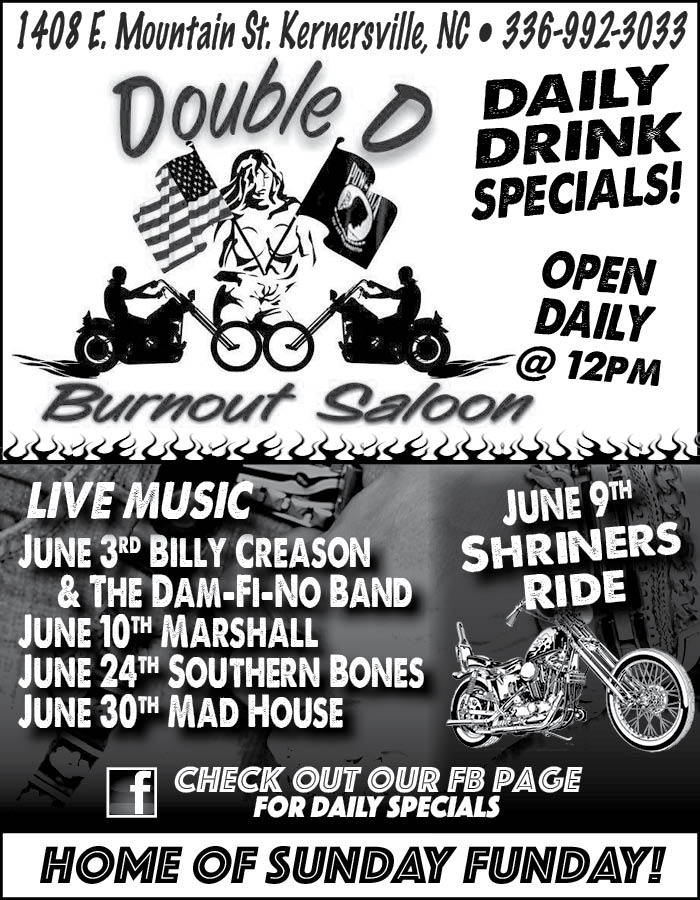https://www.facebook.com/Double-D-Burnout-Saloon
 /* styles */ JUN 9 7TH ANNUAL SETH NALL MEMORIAL SCHOLARSHIP RIDE Mt. Vernon United Methodist Church 4732 Robbins Country Rd., Trinity, NC. Reg 9:30 KSU 11 police escorted, 50/50, food, door prizes, cash prize, auction, music. Rain or shine. Benefits the Seth Nall scholarship fund at Trinity High School. Tim Nall 336-989-5000.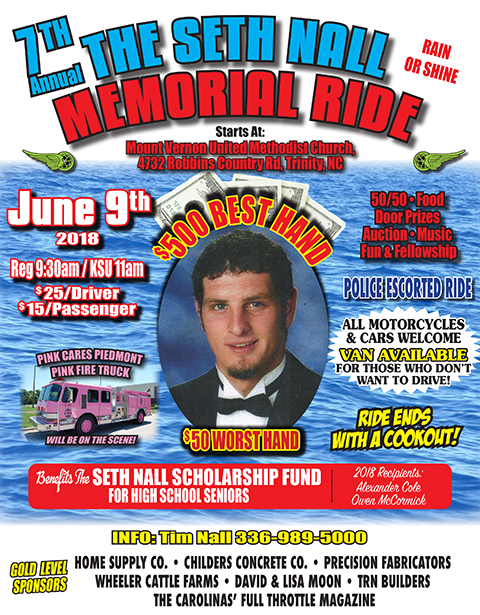/* styles */ JUN 9 GEMINI PARTY JR’S Corner Tavern 901 Greensboro Rd., High Point, NC. 2-until. 336-875-4177.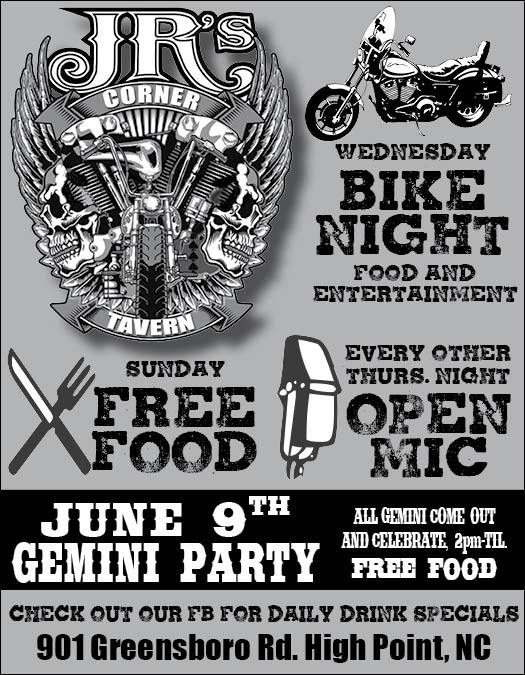www.facebook.com/JRscornertavern
 /* styles */ JUN 9 BENEFIT RIDE FOR TOMMY MCDOWELL Union Grove Community Building 1379 Union Church Rd., Asheboro, NC. KSU 12 \$20/sgl \$25/dbl, 50/50, food. Lou Parrish 336-953-1045 or Angel McDowell 336-588-1344. JUN 9 POKER RUN FOR CHARITY BY AWOL MC Richards Coffee Shop 165 N Main St., Mooresville, NC. Reg 10-1 \$15/rider \$5/pass, live music, food. Benefits our Military Veterans. Bush 704-878-5554. JUN 9 ROLLING THUNDER POKER RUN 2018 Tilley Harley-Davidson Statesville 1226 Morland Dr., Statesville, NC. Reg 9 last out 11, \$20/sgl \$25/dbl includes meal and door prizes. 50/50, door prizes. Rain or shine. Rolling Thunder NC II Sonny Deal 704-929-0477, Rhonda Deal 336-469-3415 or Mark Terry 336-480-4624.
 table div table+table+table+table+table+table+table+table+table+table+table+table+table+table+table+table+table+table+table+table+table+table+table+table+table+table+table+table+table+table div table td,table.module-29{width:100%;padding:0}table div table+table+table+table+table+table+table+table+table+table+table+table+table+table+table+table+table+table+table+table+table+table+table+table+table+table+table+table+table+table div table{width:100%;float:none;margin-left:auto;margin-right:auto;padding:0}table div table+table+table+table+table+table+table+table+table+table+table+table+table+table+table+table+table+table+table+table+table+table+table+table+table+table+table+table+table+table div table a{border:0 none;text-decoration:none}table div table+table+table+table+table+table+table+table+table+table+table+table+table+table+table+table+table+table+table+table+table+table+table+table+table+table+table+table+table+table div table img{width:100%!important;border:0 none;text-decoration:none}/* styles */
 /* styles */ JUN 9 CALEB BENFIELD BENEFIT POKER RUN Hooters 1211 13th Ave Dr. SE Hickory, NC. Reg 10:30-1 \$20/sgl \$25/dbl includes t-shirt, 50/50, food. Benefits Caleb Benfield Challenge. Sponsored by Blue Knights NCXI 828-413-9706. JUN 9 RIDE FOR READERS Freeborne’s 14300 NC Hwy 18 S., Laurel Springs, NC. KSU 10, \$25/person, food, live music, 50/50. Benefits Northwestern Regional Library. 336-835-4894. JUN 9 IN-VEST USA BENEFIT RUN Harley-Davidson of Asheville 20 Patton Cove Rd., Swannanoa, NC. Reg 10 \$20/bike. 828-581-2000. JUN 9 POKER RUN FOR MRS. BONNIE OF SCORES Jamin Leather 1222 Port Dr., Myrtle Beach, SC. KSU 12, \$20/rider \$10/pass, 50/50, raffles. 843-294-1222. JUN 9 DYNO SHOOTOUT Archie’s Custom Cycle 105 East Craft St., Swansea, SC. 12-5, door prizes, live music, food truck. 803-568-4221.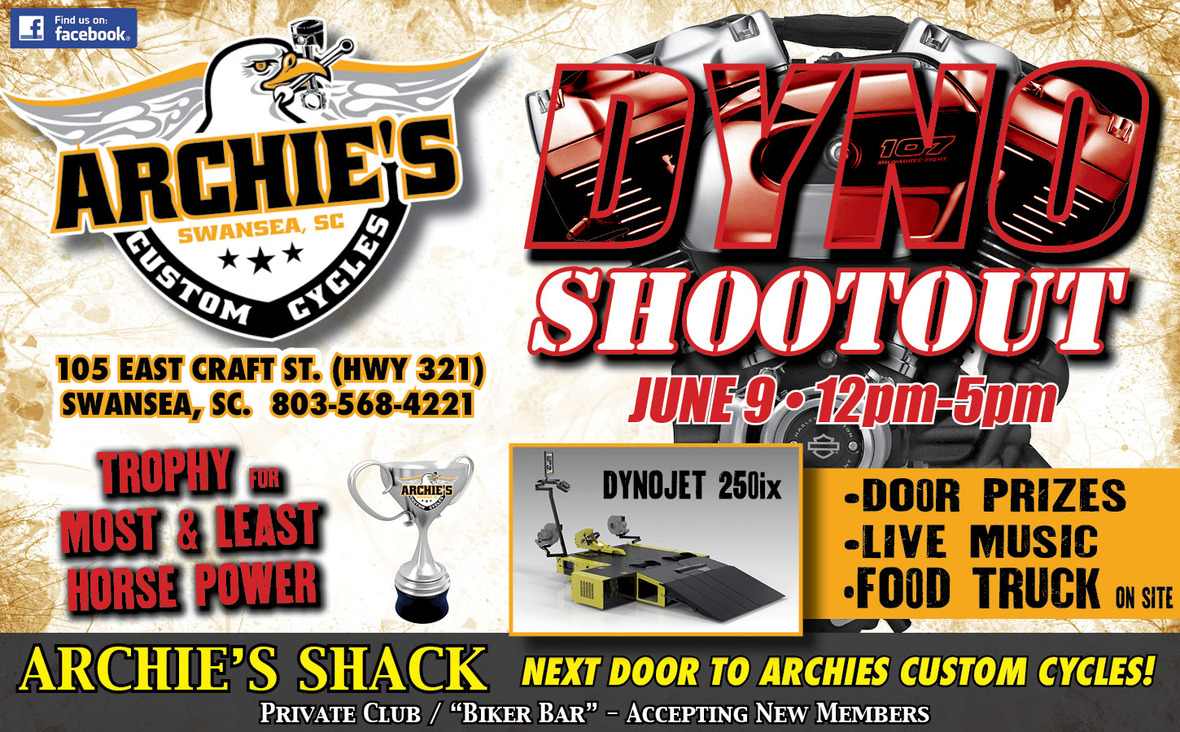# SATURDAY-SUNDAY, JUNE 9-10

 /* styles */ JUN 9-10 DAYS OF THUNDER XII Thunder Tower Harley-Davidson 190 Pontiac Business Center Dr., Columbia, SC. All day concert series both days, vendors, food, Sat. Ms.Bikini Coast Internat’l, fireworks, Sun. bike stereo sound-off, bike & car show. www.thundertowerharley.com 803-461-1121.
 table div table+table+table+table+table+table+table+table+table+table+table+table+table+table+table+table+table+table+table+table+table+table+table+table+table+table+table+table+table+table+table+table+table+table+table div table td,table.module-34{width:100%;padding:0}table div table+table+table+table+table+table+table+table+table+table+table+table+table+table+table+table+table+table+table+table+table+table+table+table+table+table+table+table+table+table+table+table+table+table+table div table{width:100%;float:none;margin-left:auto;margin-right:auto;padding:0}table div table+table+table+table+table+table+table+table+table+table+table+table+table+table+table+table+table+table+table+table+table+table+table+table+table+table+table+table+table+table+table+table+table+table+table div table a{border:0 none;text-decoration:none}table div table+table+table+table+table+table+table+table+table+table+table+table+table+table+table+table+table+table+table+table+table+table+table+table+table+table+table+table+table+table+table+table+table+table+table div table img{width:100%!important;border:0 none;text-decoration:none}/* styles */

# SUNDAY, JUNE 10

 /* styles */ JUN 10 ABATE OF SC COLUMBIA RIDERS MEETING The New Ole Place 3311 Two Notch Rd., Columbia, SC. 2pm. 803-754-5825.

# MONDAY, JUNE 11

 /* styles */ JUN 11 10TH ANNIVERSARY HONORING THEIR SERVICE MOTORCYLCE ESCORT AND PARADE Southern Wesleyan 907 Wesleyan Dr., Central, SC. Staging 11:30. Patriotic apparel is encouraged. Fly the USA, POW/MIA & US Military flags. Parade will follow the escort. Music, food, vendors. Come welcome these US Marines home. Jim Hasick 864-723-2850.
 table div table+table+table+table+table+table+table+table+table+table+table+table+table+table+table+table+table+table+table+table+table+table+table+table+table+table+table+table+table+table+table+table+table+table+table+table+table+table+table+table div table{width:100%;padding:0}table div table+table+table+table+table+table+table+table+table+table+table+table+table+table+table+table+table+table+table+table+table+table+table+table+table+table+table+table+table+table+table+table+table+table+table+table+table+table+table+table div table img{width:96.23%;padding:0;float:none}table div table+table+table+table+table+table+table+table+table+table+table+table+table+table+table+table+table+table+table+table+table+table+table+table+table+table+table+table+table+table+table+table+table+table+table+table+table+table+table+table div table td{width:100%;padding:0 1.88% 18px}/* styles */table div table+table+table+table+table+table+table+table+table+table+table+table+table+table+table+table+table+table+table+table+table+table+table+table+table+table+table+table+table+table+table+table+table+table+table+table+table+table+table+table+table div table{width:100%;padding:0}table div table+table+table+table+table+table+table+table+table+table+table+table+table+table+table+table+table+table+table+table+table+table+table+table+table+table+table+table+table+table+table+table+table+table+table+table+table+table+table+table+table div table img{width:96.23%;padding:0;float:none}table div table+table+table+table+table+table+table+table+table+table+table+table+table+table+table+table+table+table+table+table+table+table+table+table+table+table+table+table+table+table+table+table+table+table+table+table+table+table+table+table+table div table td{width:100%;padding:0 1.88% 18px}/* styles */table div table+table+table+table+table+table+table+table+table+table+table+table+table+table+table+table+table+table+table+table+table+table+table+table+table+table+table+table+table+table+table+table+table+table+table+table+table+table+table+table+table+table div table{width:100%;padding:0}table div table+table+table+table+table+table+table+table+table+table+table+table+table+table+table+table+table+table+table+table+table+table+table+table+table+table+table+table+table+table+table+table+table+table+table+table+table+table+table+table+table+table div table img{width:96.23%;padding:0;float:none}table div table+table+table+table+table+table+table+table+table+table+table+table+table+table+table+table+table+table+table+table+table+table+table+table+table+table+table+table+table+table+table+table+table+table+table+table+table+table+table+table+table+table div table td{width:100%;padding:0 1.88% 18px}/* styles */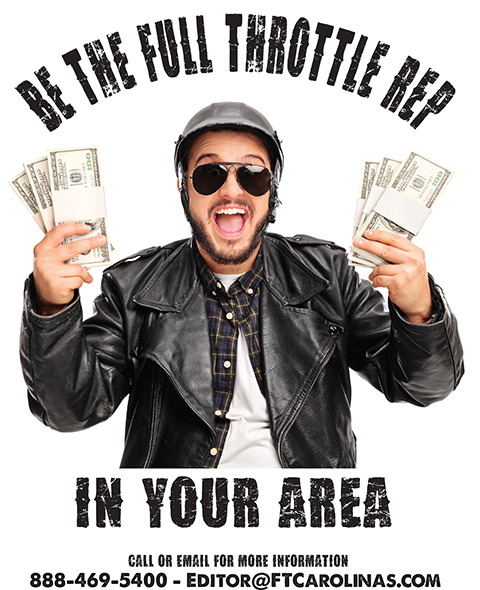table div table+table+table+table+table+table+table+table+table+table+table+table+table+table+table+table+table+table+table+table+table+table+table+table+table+table+table+table+table+table+table+table+table+table+table+table+table+table+table+table+table+table+table+table div table{width:100%;padding:0}table div table+table+table+table+table+table+table+table+table+table+table+table+table+table+table+table+table+table+table+table+table+table+table+table+table+table+table+table+table+table+table+table+table+table+table+table+table+table+table+table+table+table+table+table div table img{width:96.23%;padding:0;float:none}table div table+table+table+table+table+table+table+table+table+table+table+table+table+table+table+table+table+table+table+table+table+table+table+table+table+table+table+table+table+table+table+table+table+table+table+table+table+table+table+table+table+table+table+table div table td{width:100%;padding:0 1.88% 18px}/* styles */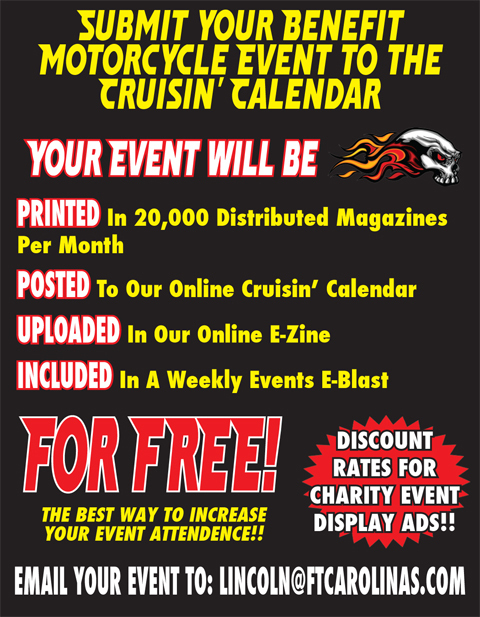Click On The Ad To Post Your Event!
 table div table+table+table+table+table+table+table+table+table+table+table+table+table+table+table+table+table+table+table+table+table+table+table+table+table+table+table+table+table+table+table+table+table+table+table+table+table+table+table+table+table+table+table+table+table+table div table{width:100%;padding:0}table div table+table+table+table+table+table+table+table+table+table+table+table+table+table+table+table+table+table+table+table+table+table+table+table+table+table+table+table+table+table+table+table+table+table+table+table+table+table+table+table+table+table+table+table+table+table div table img{width:96.23%;padding:0;float:none}table div table+table+table+table+table+table+table+table+table+table+table+table+table+table+table+table+table+table+table+table+table+table+table+table+table+table+table+table+table+table+table+table+table+table+table+table+table+table+table+table+table+table+table+table+table+table div table td{width:100%;padding:0 1.88% 18px}/* styles */# BIKE NIGHTS!

 /* styles */ Want To See Your Bike Night Listed Here? Call 336-885-5400

# MONDAY

DOUBLE D BURNOUT SALOON 1408 E. Mountain St. Kernersville, NC. 336-992-3033

SONS OF THE SOUTH 3007 1st Ave. SW, Hickory, NC. 828-855-3097

WIZARD SALOON 651 21st St. NE, Hickory, NC 828-327-8555

# TUESDAY

DOUBLE D BURNOUT SALOON 1408 E. Mountain St. Kernersville, NC. 336-992-3033

SONS OF THE SOUTH 3007 1st Ave. SW, Hickory, NC. 828-855-3097

WIZARD SALOON 651 21st St. NE, Hickory, NC 828-327-8555

# WEDNESDAY

DOUBLE D BURNOUT SALOON 1408 E. Mountain St. Kernersville, NC. 336-992-3033

JR'S CORNER TAVERN 901 Greensboro Rd. High Point, NC 336-875-4179

SONS OF THE SOUTH 3007 1st Ave. SW, Hickory, NC. 828-855-3097

WIZARD SALOON 651 21st St. NE, Hickory, NC 828-327-8555

# THURSDAY

DOUBLE D BURNOUT SALOON 1408 E. Mountain St. Kernersville, NC. 336-992-3033

LUCKY'S SALOON 1453 Industry Dr, Burlington, NC. 336-570-1050

NORTHSIDE TAVERN 50-A Wee Care Ave. Sparta, NC 336-372-4008

SHELTON'S HARLEY-DAVIDSON @Texas Steakhouse, 1st & 3rd Thursdays. 235 Outlet Center Dr. Smithfield, NC 919-938-1592

SONS OF THE SOUTH 3007 1st Ave. SW, Hickory, NC. 828-855-3097

TED’S KICKIN’ CHICKEN 4695 S. Main St., Winston-Salem NC. 336-650-0290

WIZARD SALOON 651 21st St. NE, Hickory, NC 828-327-8555

XTREME WINGS -N- THINGS 3441 Myer Lee Dr, Winston-Salem NC
336-293-4983

# FRIDAY

DOUBLE D BURNOUT SALOON 1408 E. Mountain St. Kernersville, NC. 336-992-3033

SONS OF THE SOUTH 3007 1st Ave. SW, Hickory, NC. 828-855-3097

WIZARD SALOON 651 21st St. NE, Hickory, NC 828-327-8555

# SATURDAY

DOUBLE D BURNOUT SALOON 1408 E. Mountain St. Kernersville, NC. 336-992-3033.

SONS OF THE SOUTH 3007 1st Ave. SW, Hickory, NC. 828-855-3097

WIZARD SALOON 651 21st St. NE, Hickory, NC 828-327-8555

# SUNDAY

DOUBLE D BURNOUT SALOON 1408 E. Mountain St. Kernersville, NC. 336-992-3033

SONS OF THE SOUTH 3007 1st Ave. SW, Hickory, NC. 828-855-3097

TANK TOWN TAVERN 6711 Freedom Dr., Charlotte, NC. 704-277-0268 12pm-?

TRAX TAVERN 46 E. Main St. Thomasville, NC. 2-9pm

WIZARD SALOON 651 21st St. NE, Hickory, NC 828-327-8555

 table div table+table+table+table+table+table+table+table+table+table+table+table+table+table+table+table+table+table+table+table+table+table+table+table+table+table+table+table+table+table+table+table+table+table+table+table+table+table+table+table+table+table+table+table+table+table+table+table+table+table div table{width:100%;padding:0}table div table+table+table+table+table+table+table+table+table+table+table+table+table+table+table+table+table+table+table+table+table+table+table+table+table+table+table+table+table+table+table+table+table+table+table+table+table+table+table+table+table+table+table+table+table+table+table+table+table+table div table img{width:96.23%;padding:0;float:none}table div table+table+table+table+table+table+table+table+table+table+table+table+table+table+table+table+table+table+table+table+table+table+table+table+table+table+table+table+table+table+table+table+table+table+table+table+table+table+table+table+table+table+table+table+table+table+table+table+table+table div table td{width:100%;padding:0 1.88% 18px}/* styles */# GREAT AMERICAN COOKOUT - JUNE 23

 table div table+table+table+table+table+table+table+table+table+table+table+table+table+table+table+table+table+table+table+table+table+table+table+table+table+table+table+table+table+table+table+table+table+table+table+table+table+table+table+table+table+table+table+table+table+table+table+table+table+table+table+table div table td,table.module-51{width:100%;padding:0}table div table+table+table+table+table+table+table+table+table+table+table+table+table+table+table+table+table+table+table+table+table+table+table+table+table+table+table+table+table+table+table+table+table+table+table+table+table+table+table+table+table+table+table+table+table+table+table+table+table+table+table+table div table{width:100%;float:none;margin-left:auto;margin-right:auto;padding:0}table div table+table+table+table+table+table+table+table+table+table+table+table+table+table+table+table+table+table+table+table+table+table+table+table+table+table+table+table+table+table+table+table+table+table+table+table+table+table+table+table+table+table+table+table+table+table+table+table+table+table+table+table div table a{border:0 none;text-decoration:none}table div table+table+table+table+table+table+table+table+table+table+table+table+table+table+table+table+table+table+table+table+table+table+table+table+table+table+table+table+table+table+table+table+table+table+table+table+table+table+table+table+table+table+table+table+table+table+table+table+table+table+table+table div table img{width:100%!important;border:0 none;text-decoration:none}/* styles */
 table div table+table+table+table+table+table+table+table+table+table+table+table+table+table+table+table+table+table+table+table+table+table+table+table+table+table+table+table+table+table+table+table+table+table+table+table+table+table+table+table+table+table+table+table+table+table+table+table+table+table+table+table+table div table{width:100%;padding:0}table div table+table+table+table+table+table+table+table+table+table+table+table+table+table+table+table+table+table+table+table+table+table+table+table+table+table+table+table+table+table+table+table+table+table+table+table+table+table+table+table+table+table+table+table+table+table+table+table+table+table+table+table+table div table img{width:96.23%;padding:0;float:none}table div table+table+table+table+table+table+table+table+table+table+table+table+table+table+table+table+table+table+table+table+table+table+table+table+table+table+table+table+table+table+table+table+table+table+table+table+table+table+table+table+table+table+table+table+table+table+table+table+table+table+table+table+table div table td{width:100%;padding:0 1.88% 18px}/* styles */## The Carolinas' Full Throttle Magazine Virtual Bike Show!

Vote On Line For Your Favorite Bike On Our Website. The Bikes That Receive The Top 3 Most Votes Will Be Featured In The Carolinas' Full Throttle Magazine!!!

Check it out NOW!

 table div table+table+table+table+table+table+table+table+table+table+table+table+table+table+table+table+table+table+table+table+table+table+table+table+table+table+table+table+table+table+table+table+table+table+table+table+table+table+table+table+table+table+table+table+table+table+table+table+table+table+table+table+table+table+table div table{width:100%;padding:0}table div table+table+table+table+table+table+table+table+table+table+table+table+table+table+table+table+table+table+table+table+table+table+table+table+table+table+table+table+table+table+table+table+table+table+table+table+table+table+table+table+table+table+table+table+table+table+table+table+table+table+table+table+table+table+table div table img{width:96.23%;padding:0;float:none}table div table+table+table+table+table+table+table+table+table+table+table+table+table+table+table+table+table+table+table+table+table+table+table+table+table+table+table+table+table+table+table+table+table+table+table+table+table+table+table+table+table+table+table+table+table+table+table+table+table+table+table+table+table+table+table div table td{width:100%;padding:0 1.88% 18px}/* styles */# Read The Carolinas' Full Throttle Magazine ONLINE!!!

 table div table+table+table+table+table+table+table+table+table+table+table+table+table+table+table+table+table+table+table+table+table+table+table+table+table+table+table+table+table+table+table+table+table+table+table+table+table+table+table+table+table+table+table+table+table+table+table+table+table+table+table+table+table+table+table+table+table div table{width:100%;padding:0}table div table+table+table+table+table+table+table+table+table+table+table+table+table+table+table+table+table+table+table+table+table+table+table+table+table+table+table+table+table+table+table+table+table+table+table+table+table+table+table+table+table+table+table+table+table+table+table+table+table+table+table+table+table+table+table+table+table div table img{width:96.23%;padding:0;float:none}table div table+table+table+table+table+table+table+table+table+table+table+table+table+table+table+table+table+table+table+table+table+table+table+table+table+table+table+table+table+table+table+table+table+table+table+table+table+table+table+table+table+table+table+table+table+table+table+table+table+table+table+table+table+table+table+table+table div table td{width:100%;padding:0 1.88% 18px}/* styles */table div table+table+table+table+table+table+table+table+table+table+table+table+table+table+table+table+table+table+table+table+table+table+table+table+table+table+table+table+table+table+table+table+table+table+table+table+table+table+table+table+table+table+table+table+table+table+table+table+table+table+table+table+table+table+table+table+table+table div table,table.module-57{width:26.23%;float:right;padding:0}table div table+table+table+table+table+table+table+table+table+table+table+table+table+table+table+table+table+table+table+table+table+table+table+table+table+table+table+table+table+table+table+table+table+table+table+table+table+table+table+table+table+table+table+table+table+table+table+table+table+table+table+table+table+table+table+table+table+table div table a{border:0 none;text-decoration:none}table div table+table+table+table+table+table+table+table+table+table+table+table+table+table+table+table+table+table+table+table+table+table+table+table+table+table+table+table+table+table+table+table+table+table+table+table+table+table+table+table+table+table+table+table+table+table+table+table+table+table+table+table+table+table+table+table+table+table div table img{width:100%!important;border:0 none;text-decoration:none}table div table+table+table+table+table+table+table+table+table+table+table+table+table+table+table+table+table+table+table+table+table+table+table+table+table+table+table+table+table+table+table+table+table+table+table+table+table+table+table+table+table+table+table+table+table+table+table+table+table+table+table+table+table+table+table+table+table+table div table td{width:100%;padding:0 0 20px 20px}/* styles */ The Carolinas' Full Throttle Magazine is on Facebook "Like" us on Facebook for daily bike night info, pictures, videos, and other biker news! Click here for our Facebook page!
 table div table+table+table+table+table+table+table+table+table+table+table+table+table+table+table+table+table+table+table+table+table+table+table+table+table+table+table+table+table+table+table+table+table+table+table+table+table+table+table+table+table+table+table+table+table+table+table+table+table+table+table+table+table+table+table+table+table+table+table div table{width:100%;padding:0}table div table+table+table+table+table+table+table+table+table+table+table+table+table+table+table+table+table+table+table+table+table+table+table+table+table+table+table+table+table+table+table+table+table+table+table+table+table+table+table+table+table+table+table+table+table+table+table+table+table+table+table+table+table+table+table+table+table+table+table div table img{width:96.23%;padding:0;float:none}table div table+table+table+table+table+table+table+table+table+table+table+table+table+table+table+table+table+table+table+table+table+table+table+table+table+table+table+table+table+table+table+table+table+table+table+table+table+table+table+table+table+table+table+table+table+table+table+table+table+table+table+table+table+table+table+table+table+table+table div table td{width:100%;padding:0 1.88% 18px}/* styles */# GET YOUR FULL THROTTLE GEAR!

Official Carolinas' Full Throttle Magazine Shirts, Koozies, Shotglasses!

# FREE SHIPPING!!!

Check It Out!

 Like   Tweet